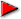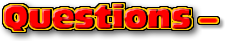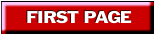ChaptersPage 1This text is meant to accompany class discussions. It is not everything there is to know about the basics of electricity. It is meant as a  prep for class. More detailed notes and examples are given in the class notes, presentations, and demonstrations (click here.)Click for the questions that go with this reading Introduction In the typical high school math class, every quantity is represented by a number. ...Only the number. In order for math to model the physical world, there needs to be more -and there is. In physics, often it is not enough to mention only a number but the concept of direction needs to be added to the description. This is done by the use of vectors. A vector is a term used to describe a measurement or concept that is described by a number and a direction. Magnitude describes the size of a measurement. When scientists use vectors they refer to the number part as the magnitude. For example, an Earthquake of magnitude 8.9 is bigger and has more destructive capabilities than an earthquake of magnitude 3. A category 4 hurricane can do more damage than a category 1 hurricane. This is because the magnitude of the winds in a category 4 are larger than the magnitude of the winds in a category 1 hurricane. Imagine your house on a map. Imagine your school on the same map and an arrow pointing from your house to your school. This arrow is a vector. The vector is described by two characteristics: Magnitude and DirectionThe length of the arrow is the magnitude. It is always a positive number. When someone asks you, "How far away do you live from school?" You might answer a 1/2 mile. "1/2" is the magnitude. It describes how away you live.But by only giving this distance, you are not giving very precise directions because you could live anywhere along a circle with a radius of 1/2 mile, see below. If you describe your home as being 1/2 mile from the physics classroom at an angle 15.2° west of pointing due south, then you have given a precise indication of where live. This is because you have given a vector, (with magnitude and direction.)A quantity that is described ONLY by a number is called scaler. The answer of 1/2 mile is a scaler. In physics, a vector is better because it succinctly describes two measurements, magnitude and direction. The answer of 1/2 mile from the physics classroom at an angle 15.2° west of pointing due south gives more precision to the response.by Tony Wayne ...(If you are a teacher, please feel free to use these resources in your teaching.)